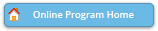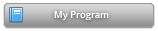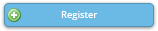Sessions Were Renumbered as of May 19.

Legend:
CC-W = McCormick Place Convention Center, West Building,   CC-N = McCormick Place Convention Center, North Building
H = Hilton Chicago,   UC= Conference Chicago at University Center
* = applied session       ! = JSM meeting theme

#### Activity DetailsTweet CE_09C Sun, 7/31/2016, 8:30 AM - 5:00 PM CC-W471 Regression Modeling Strategies (ADDED FEE) — Professional Development Continuing Education Course ASA , Biometrics Section All standard regression models have assumptions that must be verified for the model to have power to test hypotheses and be able to predict accurately. Of the principal assumptions (linearity, additivity, distributional), this course will emphasize methods for assessing and satisfying the first two. Practical but powerful tools are presented for validating model assumptions and presenting model results. This course provides methods for estimating the shape of the relationship between predictors and response using the widely applicable method of augmenting the design matrix using restricted cubic splines. Even when assumptions are satisfied, over-fitting can ruin a model's predictive ability for future observations. Methods for data reduction will be introduced to deal with the common case in which the number of potential predictors is large compared to the number of observations. Methods of model validation (bootstrap and cross validation) will be covered, as will auxiliary topics such as modeling interaction surfaces, efficiently using partial covariable data by using multiple imputation, variable selection, overly influential observations, collinearity, and shrinkage. The methods covered will apply to almost any regression model, including ordinary least squares, logistic regression models, ordinal regression, quantile regression, longitudinal data analysis, and survival models. Instructor(s): Frank Harrell, Vanderbilt University School of Medicine